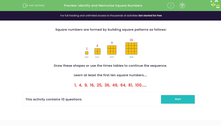# Identify and Memorise Square Numbers

In this worksheet, students will identify and memorise square numbers.Key stage:  KS 2

Curriculum topic:   Number: Multiplication and Division

Curriculum subtopic:   Recognise Square/Cube Numbers

Popular topics:   Multiplication worksheets, Square Numbers worksheets

Difficulty level:#### Worksheet Overview

Square numbers are formed by building square patterns as follows:Draw these shapes or use the times tables to continue the sequence.

Square numbers are formed when we multiply a number by itself.

Here are the first ten square numbers:

1 x 1 = 1

2 x 2 = 4

3 x 3 = 9

4 x 4 = 16

5 x 5 = 25

6 x 6 = 36

7 x 7 = 49

8 x 8 = 64

9 x 9 = 81

10 x 10 = 100

Learn at least the first ten square numbers, if you possibly can!

1,  4,  9,  16,  25,  36,  49,  64,  81,  100.....

Why not write them down a few times and then test yourself by doing it again, without looking?!### What is EdPlace?

We're your National Curriculum aligned online education content provider helping each child succeed in English, maths and science from year 1 to GCSE. With an EdPlace account you’ll be able to track and measure progress, helping each child achieve their best. We build confidence and attainment by personalising each child’s learning at a level that suits them.

Get started